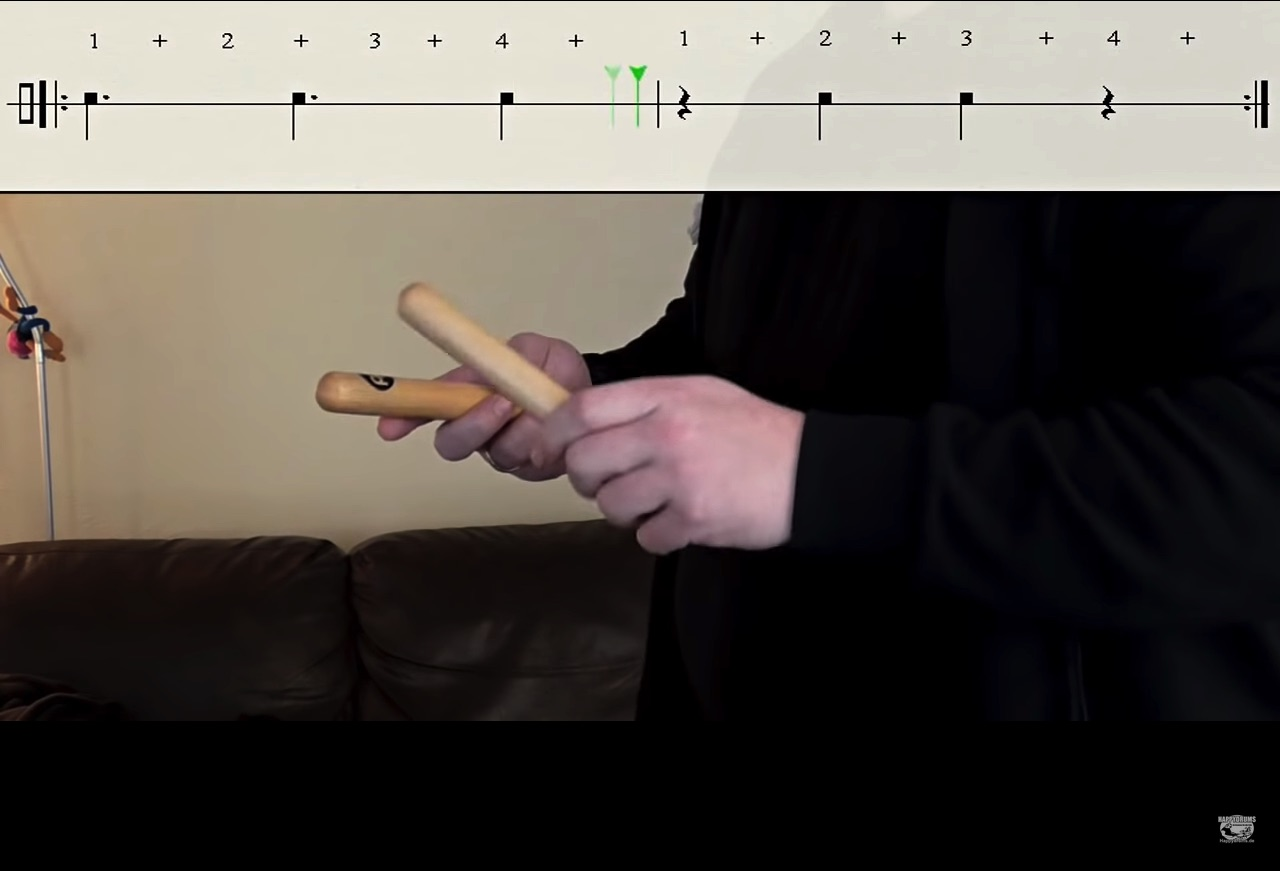Section14.6The 3–2 Son Clave

The 3–2 son clave (and the 2–3 son clave) are essential rhythmic elements in Afro-Cuban music. In this section we will see how cross rhythms implied by the 3–2 son clave can be viewed as a possible source of cross rhythms in popular music. Here is a video demonstration of a 3–2 son clave.Here is rhythmic notation of a 3–2 son clave. Notice there are three attacks in the first measure and 2 attacks in the second bar.The rhythm underlying the first bar is 3+3+2.The 3–2 son clave has also been called the “Bo Diddley Beat” because of its use by Bo Diddley in the 1950s. Look at the rhythms shown above as you listen to the following examples.

Subsection14.6.1The 3+3+2 Rhythm

In the examples below, only the first bar of the 3–2 son clave is used. Below, the 3+3+2 rhythm is shown in both eighth notes and sixteenth notes. The sixteenth-note version is known as tresillo.The example below shows the sixteenth-note subdivisions in the 3+3+2 rhythm.Below are different ways of notating the sixteenth–note version 3+3+2 rhythm.The first example, by the Rebirth Brass Band, uses a “second line” drumming pattern, which is associated with second line parades in New Orleans.

The well-known introduction to “Eye of the Tiger” uses the 3+3+2 rhythm.

In the following example from Cyndi Lauper's “Girls Just Want to Have Fun,” the lead electric guitar riff emphasizes a 3+3+2 rhythm in 16th notes on each downbeat.Figure 14.6.8. Robert Hazard, “Girls Just Want to Have Fun” (1983)

Below is an example showing the 3+3+2 rhythm (in 16th notes) immediately repeated. The rhythm is shown in two possible notations below.The following three examples all use the (3+3+2)+(3+3+2) rhythm as a rhythmic ostinato or groove.

Notice that this progression is from the Best-Seller progression.

This rhythm also occurs in the following classical piece by Ligeti.

The following examples have the (3+3+2)+(3+3+2) rhythm in 8th notes.Subsubsection14.6.1.1Habanera and Reggaeton

The reggaeton beat is built from a 3+3+2 rhythm. We will see a relationship between the reggaeton beat with the habanera. First, let us examine the famous “Habanera” bass line from the opera Carmen.Figure 14.6.16. Georges Bizet, Carmen, Habanera (“L'amour est un oiseau rebelle”) (1870)

Compare the habanera pattern above to the reggaeton beat below, notated for bass drum and snare drum.Compare the “reggaeton” rhythm to the 3+3+2 of the first bar of a 3–2 son clave (refer to the second measure in the example below).Here are examples of songs with a reggaeton beat.

Notice that the progression for “Despacito” comes from the Best-Seller progression.

In the next two sections, we will examine expansions of the 3+3+2 rhythm

Subsection14.6.23+3+3+3+2+2

In this section, we will see each number in the 3+3+2 pattern repeated, generating the 3+3+3+3+2+2 pattern.Listen for the 3+3+3+3+2+2 rhythm in the following examples.

Subsection14.6.38 Groups of 3 Plus 4 Groups of 2

the 3+3+2 pattern can also be expanded to 8 groups of 3 sixteenth notes followed by 4 groups of 2 sixteenth notes, as shown in the example below.Listen for this rhythm in the examples below.

Subsubsection14.6.1Other Combinations of 3s and 2s

You will also find other combinations of 3s and 2s (or 3s and 4s).Figure 14.6.29. Stefan Gordy, Skyler Gordy, David Listenbee, Peter Schroeder, “Party Rock Anthem” (2011)

Notice that this progression is from the i–VII–VI–VII progression. The rhythm in the above example is dissected below.Listen for the 3+3+4+3+3 rhythm in the following examples.

One also finds the 3+3+4+3+3 pattern in the second bar of “Tik Tok,” an example from earlier in the chapter.Figure 14.6.32. Kesha Sebert, Lukasz Gottwald, Benny Blanco, “Tik Tok” (2009)

Notice that this progression comes from a rotation of the i–VII–VI–VII progression.